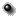OMNIWeb Plus Browser
Interface to produce plots, listings or output files
ACE SWICS 12min Flux Ratio

Time span: 1998-01-28 to 2011-12-31

Enter start and stop dates: year,month,day(YYYYMMDD) or year, day_of_year(YYYYDOY)
Start time    Stop time
Units of [particles]/([cm^2]*[Sr]*[sec]*[MeV/nucleon]>Select any two fluxes (X and Y) and get ratio: R=X/Y
Fluxes   (Numbers below are energies in MeV/n)
Protons(H)
 1.583e-04 X Y 1.701e-04 X Y 1.828e-04 X Y 1.964e-04 X Y 2.110e-04 X Y 2.267e-04 X Y 2.436e-04 X Y 2.617e-04 X Y 2.812e-04 X Y 3.021e-04 X Y 3.246e-04 X Y 3.487e-04 X Y 3.747e-04 X Y 4.026e-04 X Y
 4.325e-04 X Y 4.647e-04 X Y 4.993e-04 X Y 5.364e-04 X Y 5.764e-04 X Y 6.193e-04 X Y 6.654e-04 X Y 7.149e-04 X Y 7.681e-04 X Y 8.252e-04 X Y 8.867e-04 X Y 9.527e-04 X Y 1.024e-03 X Y 1.100e-03 X Y 1.182e-03 X Y
 1.270e-03 X Y 1.364e-03 X Y 1.466e-03 X Y 1.575e-03 X Y 1.692e-03 X Y 1.818e-03 X Y 1.953e-03 X Y 2.098e-03 X Y 2.254e-03 X Y 2.422e-03 X Y 2.603e-03 X Y 2.796e-03 X Y 3.004e-03 X Y 3.228e-03 X Y 3.468e-03 X Y
 3.726e-03 X Y 4.004e-03 X Y 4.302e-03 X Y 4.966e-03 X Y 5.732e-03 X Y 6.618e-03 X Y 7.639e-03 X Y 8.818e-03 X Y 1.018e-02 X Y 1.175e-02 X Y 1.357e-02 X Y 1.566e-02 X Y 1.808e-02 X Y 2.087e-02 X Y
Alpha(He)
 6.570e-04 X Y 7.059e-04 X Y 7.585e-04 X Y 8.149e-04 X Y 8.756e-04 X Y 9.407e-04 X Y 1.011e-03 X Y 1.086e-03 X Y 1.167e-03 X Y 1.254e-03 X Y 1.347e-03 X Y 1.447e-03 X Y 1.555e-03 X Y 1.671e-03 X Y
 1.795e-03 X Y 1.929e-03 X Y 2.072e-03 X Y 2.226e-03 X Y 2.392e-03 X Y 2.570e-03 X Y 2.761e-03 X Y 2.967e-03 X Y 3.188e-03 X Y 3.425e-03 X Y 3.680e-03 X Y 3.954e-03 X Y 4.248e-03 X Y 4.564e-03 X Y 4.904e-03 X Y
 5.269e-03 X Y 5.661e-03 X Y 6.082e-03 X Y 6.535e-03 X Y 7.021e-03 X Y 7.544e-03 X Y 8.105e-03 X Y 8.708e-03 X Y 9.356e-03 X Y 1.005e-02 X Y 1.080e-02 X Y 1.160e-02 X Y 1.247e-02 X Y 1.340e-02 X Y 1.439e-02 X Y
 1.546e-02 X Y 1.662e-02 X Y 1.785e-02 X Y 2.061e-02 X Y 2.379e-02 X Y 2.746e-02 X Y 3.170e-02 X Y 3.660e-02 X Y 4.225e-02 X Y 4.877e-02 X Y 5.630e-02 X Y 6.499e-02 X Y 7.503e-02 X Y 8.661e-02 X Y

Select an activity
 Plot data(for non-zero values only) Retrieve data Plot data   Ratio, X and Y Ratio only Show uncertanties List data Create fileAdvanced plot selections (optional)
 Y-axis Scale: Linear Log10 Character size(0.5-2.0): Plot Symbol: Asterisk None Plus Period Diamond Triangle Square X Symbol Size(0.1-4.0): Image size (pixels) X: Y:

 If you have any questions/comments about the OMNWeb Plus, contact Dr. Natalia Papitashvili,Mail Code 672, NASA/Goddard Space Flight Center, Greenbelt, MD 20771

NASA Official: Robert Candey (Robert.M.Candey@nasa.gov), Head of the Space Physics Data Facility